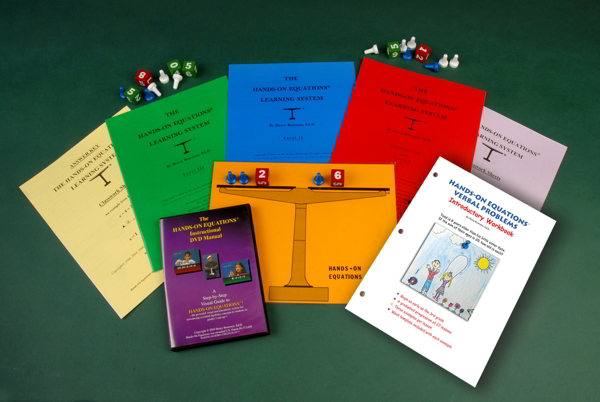### Hands-On Equations 1

Using the balance, pawns, and number cubes. Includes using guess and check to find the value of x as well as how to record and check answers.### Hands-On Equations 2

Transitions from pictures of the pawn to using an x in equations.### Hands-On Equations 3

Introduces the idea that you can remove equal amounts of pawns from each side as a legal move.### Hands-On Equations 4

Introduces that you can remove equal whole numbers from each side as a legal move.Subtraction